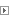These are my predictions_ i_m253_1313=58% i_m253_nd=11% i_m253_as7e=7% i_l22_n=7% i_m253_asgen=6% i_m253_as2=4% i_l22_bothnia=4% question where does this place me on the phylogenic tree?Reply With Quote

2. i_m253_1313=AS1313 Most likely it would put you as follows: M253>DF29>Z58>Z138 Do you have the following values? DYS389i=12, DYS389ii=29, DYS607=13, DYS487=15, DYS557=15 or greater.Reply With Quote

####Posting Permissions

• You may not post new threads
• You may not post replies
• You may not post attachments
• You may not edit your posts
•